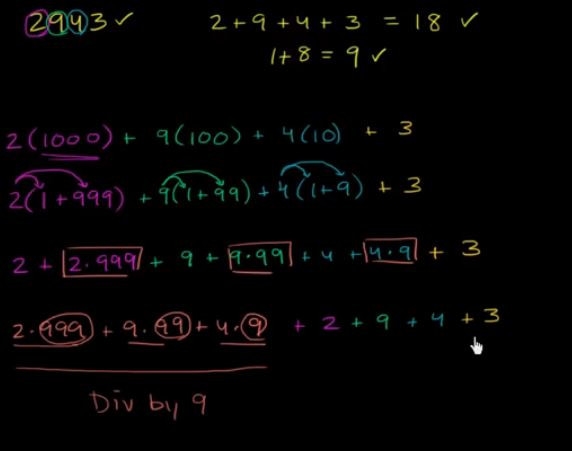DAY 7
0

# 今日kata

Given n, take the sum of the digits of n. If that value has more than one digit, continue reducing in this way until a single-digit number is produced. This is only applicable to the natural numbers.

``````    16  -->  1 + 6 = 7
942  -->  9 + 4 + 2 = 15  -->  1 + 5 = 6
132189  -->  1 + 3 + 2 + 1 + 8 + 9 = 24  -->  2 + 4 = 6
493193  -->  4 + 9 + 3 + 1 + 9 + 3 = 29  -->  2 + 9 = 11  -->  1 + 1 = 2
``````

## 構想&解法

``````function digital_root(n) {
while(n>9){
arr=n.toString().split('')
n=arr.map(item=>parseInt(item)).reduce((acc,cur)=>acc+cur)
}
return n;
}
``````

## 其他解法觀摩

``````function digital_root(n) {
return (n - 1) % 9 + 1;
}
``````

• 要知道的是 除以9的特性:
通常要快速`判斷一個數字是不是9的倍數`，要將該數字的各個位數相加，如果最後得到的結果為9的倍數，即該數字為9的倍數。EX:57132=5+7+1+3+2=18，1+8=9，9能被9整除，故57132為9的倍數
`任何一個整數若能被9整除，則該數的各個位數相加也能被9整除`
演化成`任何一個整數除以9的餘數一定等於該數的各個位數相加結果除以9的餘數`
忘記這個以前常用的性質也沒關係。

• 推薦另一個影片:說明9的整除The why of the 9 divisibility rule
其中節錄影片重點:為什麼個位數相加可以拿來判斷是否被9整除

``````    2934 可以表示成
= 2 x (1000) + 9 x (100) + 3 x (10) + 4
= 2 x (1+999) + 9 x (1+99) + 3 x (1+9) + 4
= 2x999 + 2 + 9x99 +9 + 3x9 + 3 + 4
=2x999 + 9x99 + 3x9 + 2 + 9 + 3 + 4
2+9+3+4=18 即為2934除以9的餘數
``````2934-->18-->9

(2934-1)=2933--->17-->8，8%9=8，8+1=9

``````function digital_root(n) {
return (n - 1) % 9 + 1;
}
``````